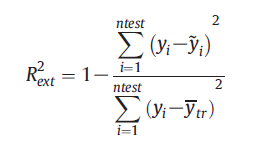# Clarification on the functioning of the Model Acceptability Criteria node

Hi novamechanics staff and users,

As another user pointed out time ago, I'm confused about the input of Model Acceptability Criteria node (https://tech.knime.org/forum/enalos-nodes/model-acceptibility-crieteria). In the node description the required input are:

• 0 Values for the dependent variable, predicted by the model (ypred).

• 1 Values for the dependent variable for the test set (yexp).

• 2 Values for the dependent variable for the training set (ytr)

Input 1 and 2 should correspond to the (experimentally measured) dependent variable for the (external) test set and for the training set, respectively. To what deal with input 0 instead, in a first moment I thought it should contain all the values predicted by the model (external set + test set) but as it is pointed out by an error from the node “Port 1 and 2 should have equal vector length”, so the only way to make the node works is by introducing in input 0 the predicted values of the (external) test set. Anyway, if this is the case, I don't understand how can you calculate the parameter that in the original paper (by Tropsha and co-workers) is called q^2 and you call as Rcvext^2 (that should be > 0.5 for the model to be reliable). According to the original paper the q^2 parameter is the (training set) cross-validated (CV) determination coefficient and to my knowledge this cannot be calculated if the CV predicted values of the training set are not provided as input.

Could you please comment on this to clarify the node functioning?

Gio

Hi,

At:

0 Values for the dependent variable, predicted by the model (ypred).

 0 Values for the dependent variable, predicted by the model (ypred). ( test set, equal lenght with input 1) 1 Values for the dependent variable for the test set (yexp). ( test set experimental values, equal lenght with input 0) 2 Values for the dependent variable for the training set (ytr). (training set experimental values)

The prediction of the test set (not the experimental values of the test set)

Rcvext^2 External cross validation  is not same with with q^2 (cross validation)Please see the following papers for the relevant equations:

A. Tropsha et al. (QSAR Comb. Sci. 22 (2003) 69-77 & Mol. Inf.  2010, 29, 476-488) (link)

Melagraki, G., Afantitis, A. “Enalos KNIME nodes: Exploring corrosion inhibition of steel in acidic medium” (2013) Chemometrics and Intelligent Laboratory Systems, 123, pp. 9-14. (link)

If you need any further clarification (for a faster answer) please also send an email to knime[at]novamechanics[dot]com

Hi Novamechanics,

Thank you for the clarification. I revised the equations from the original publication and now it's clear.

Nevertheless I have an additional doubt on one of the acceptability criteria, namely "abs(R0^2-R'0^2)". In the one of the original authors publications (i.e. A. Tropsha, Mol. Inf. 2010, 29, 476-488) the value for this criteria is meant to be < 0.3, while on the “results view” of the node is set to be < 0.1. Please, can you comment on this?